# 计算内容热度的算法解释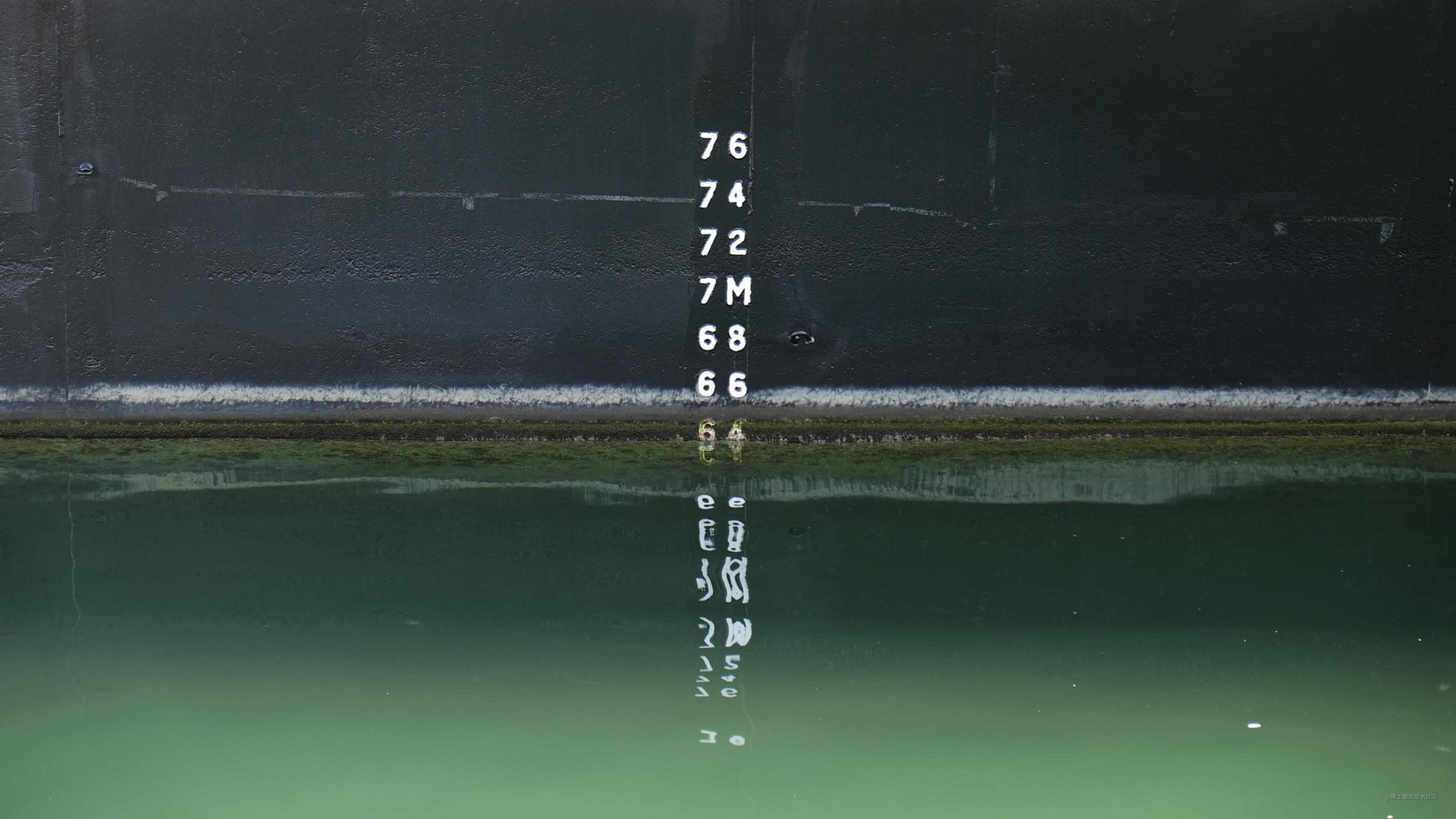## 定义一个内容的热度

$H = \frac{W + I}{(T+1)^{G}}$

• $H$：内容的热度
• $W$：内容质量的数值表达，例如一篇文章质量可以定义成文章的点赞数，也可以根据产品需要定义为其他
• $I$：新内容的初始质量，具体含义后续解释中会理解
• $G$：重力 Gravity，或者说是一个内容变得不再热门的速度，重力越大，一个内容刷新的就越快
• $T$：时间

### 1）热度$H$与时间$T$的关系

• $W$：100
• $I$：10
• $G$：1.5
• $T$：时间

$H = \frac{100+10}{(T+1)^{1.5}}$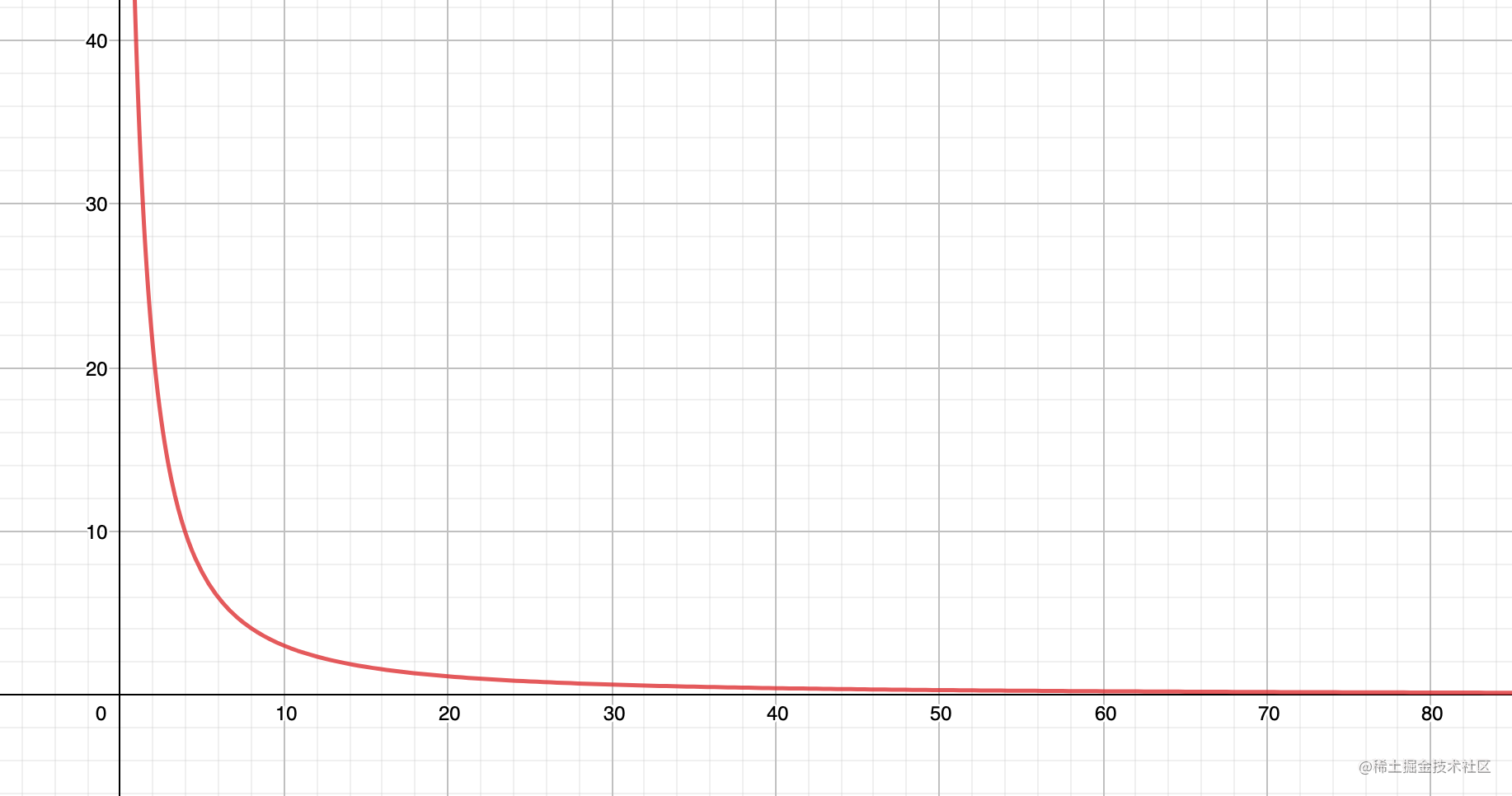### 2）热度$H$与初始质量$I$、时间$T$的关系

• $W$：0
• $I$：[2, 4, 6]
• $G$：1.5
• $T$：时间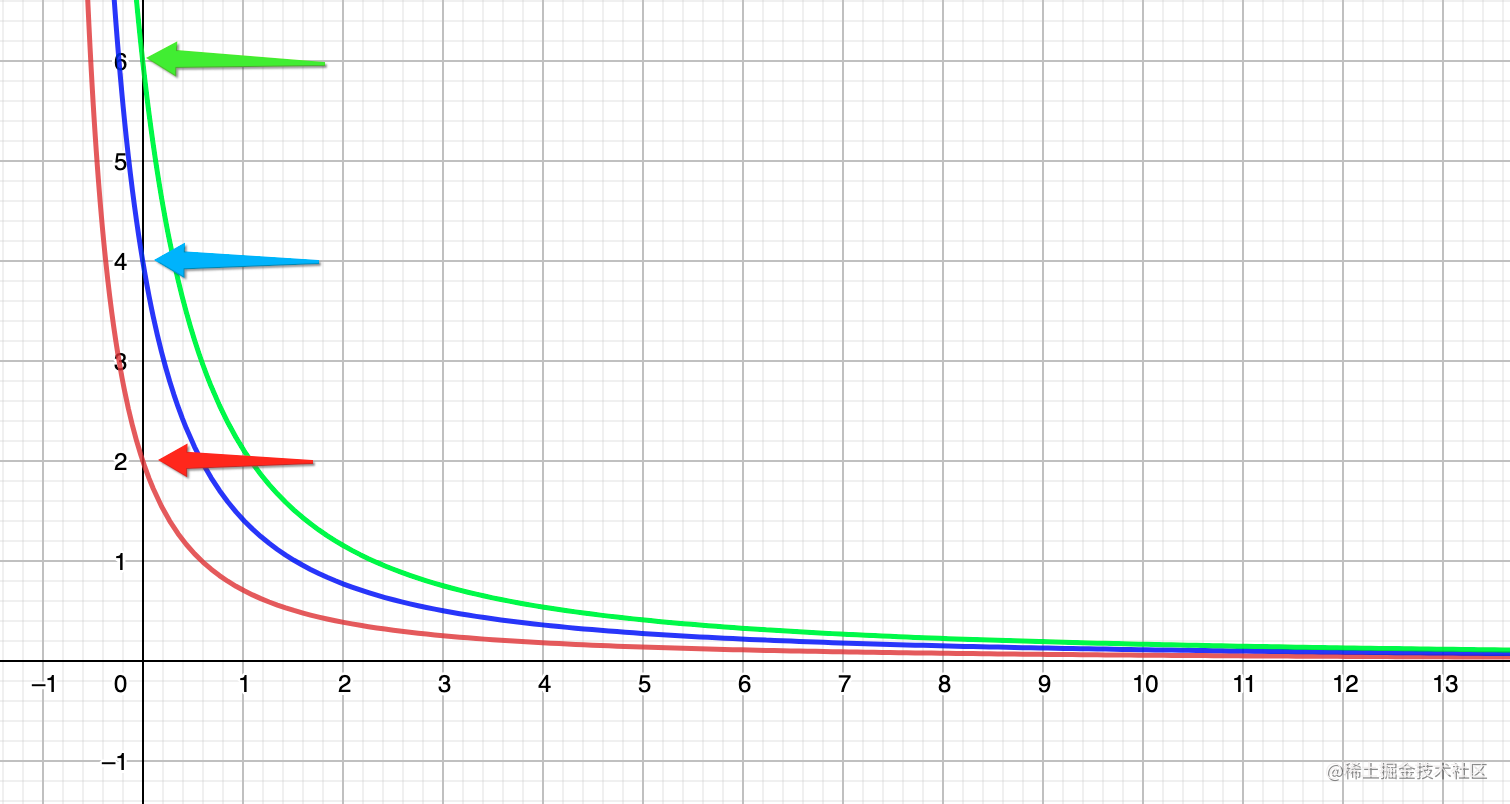### 3）热度$H$与初始质量$I$、重力$G$、时间$T$的关系

• $W$：0
• $I$：[2, 4, 6]
• $G$：[1.5, 1.2, 1.8]
• $T$：时间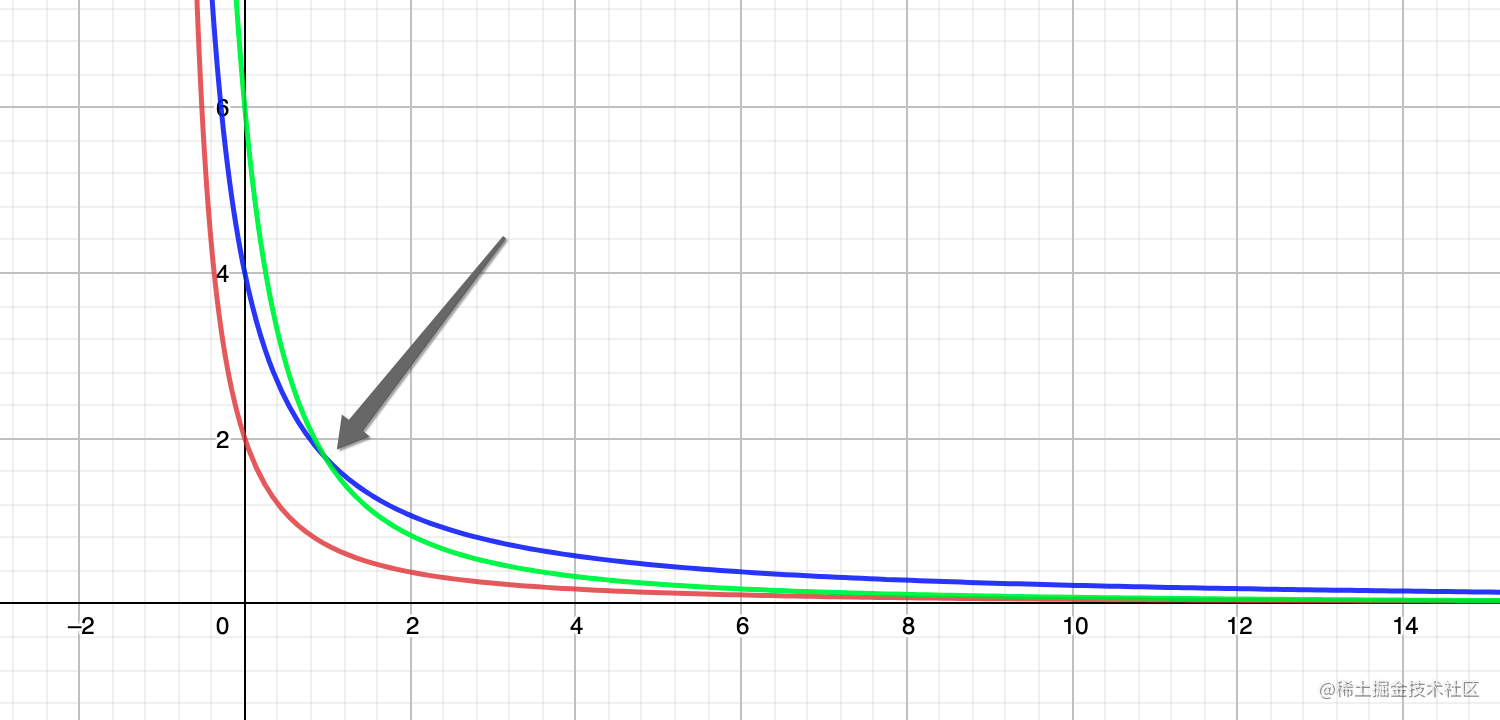### 4）热度$H$与质量$W$、初始质量$I$、重力$G$、时间$T$的关系

• $W$：[90, 190, 90]
• $I$：10
• $G$：[1.5, 1.5, 1.8]
• $T$：时间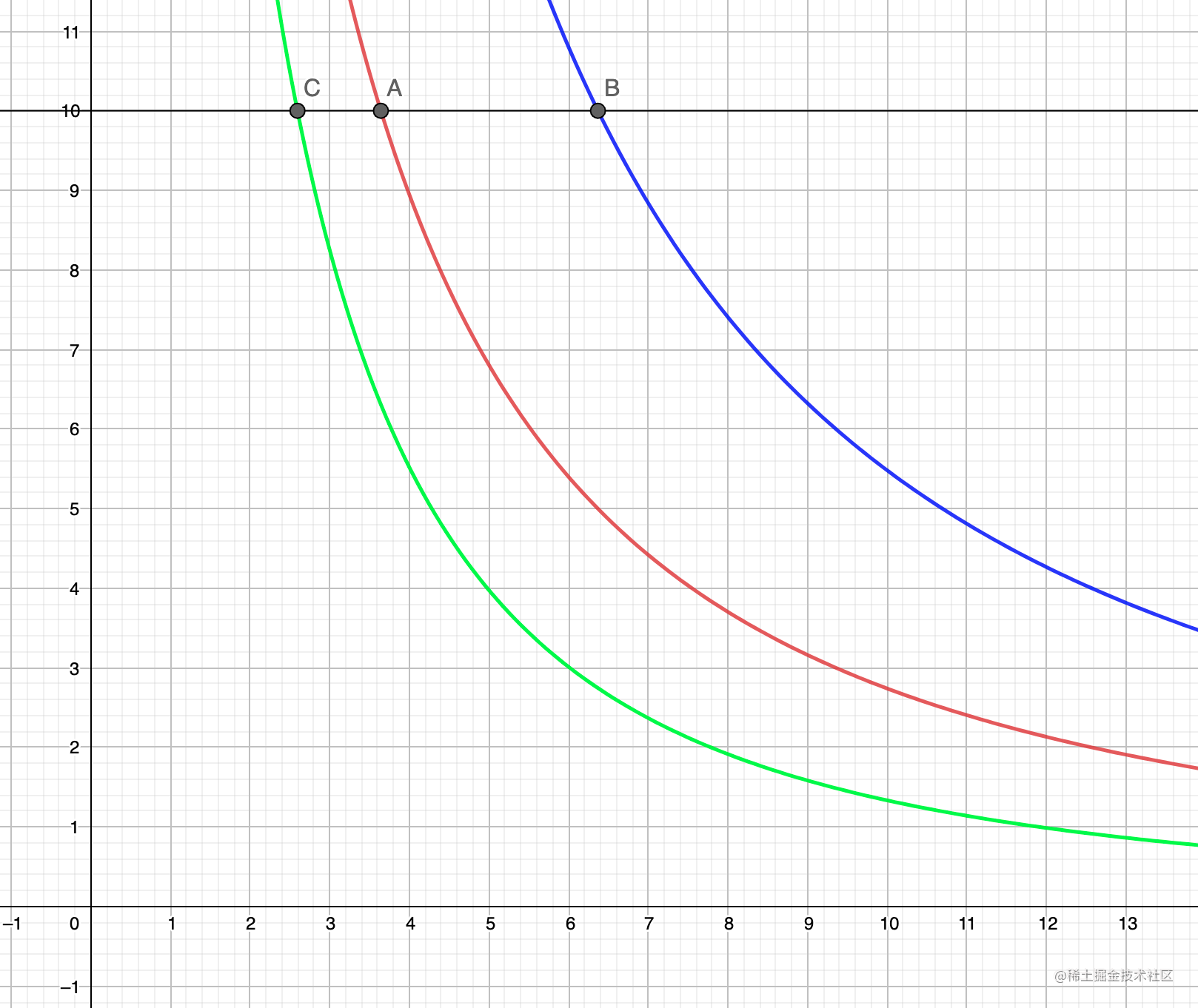• $A$$W=90$$G=1.5$$I=10$的交点

• $B$$W=190$$G=1.5$$I=10$的交点
• $C$$W=90$$G=1.8$$I=10$的交点

1. 初始质量 $I$ 越高，老内容的热度更快的被最新生产的内容超越
2. 一个内容的质量 $W$ 越高，此内容热度高于新内容的时间越长
3. 重力 $G$ 越高，内容热度衰减越快，老内容的热度更快被新生产的内容超越

### 5）质量数值$W$与时间$T$线性正相关的假设

$W(T) = c\times{T}$

$c$ 代表了一个新内容获得质量 $W$ 的速度，也就是一个新内容获得点赞的速度，那么

• $W$$c\times{T}$
• $I$：10
• $G$：1.5
• $T$：时间

$H = \frac{c\times{T}+10}{(T+1)^{1.5}}$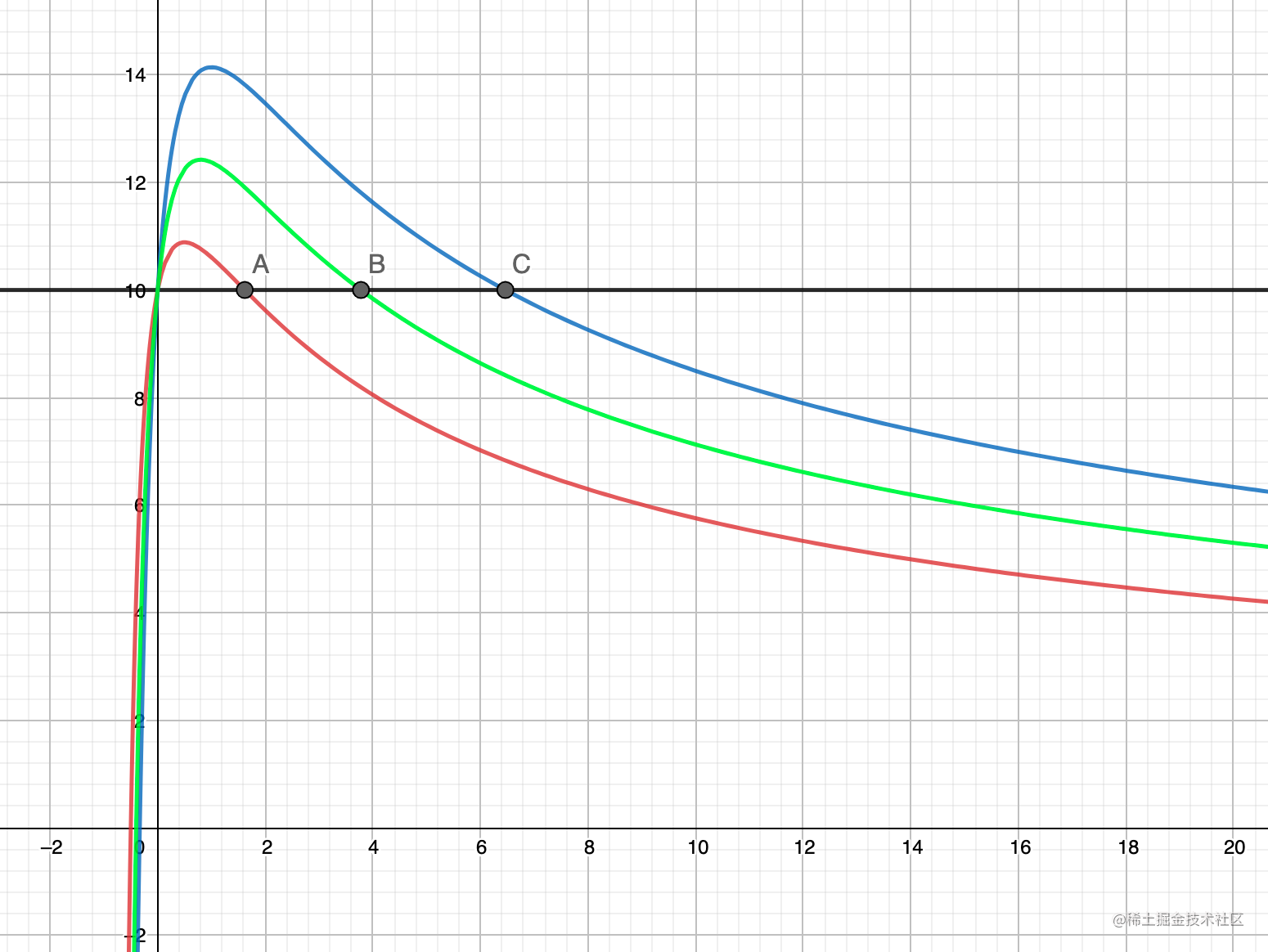• $A$$W=20\times{T}$$I=10$的交点
• $B$$W=25\times{T}$$I=10$的交点
• $C$$W=30\times{T}$$I=10$的交点

### 6）质量数值$W$与时间$T$对数正相关的假设

$W(T) = c\times{\log_{d}{(T+1)}}$

• $c$：获得点赞的速度
• $d$：随着时间迁移，获得点赞速度开始衰减的系数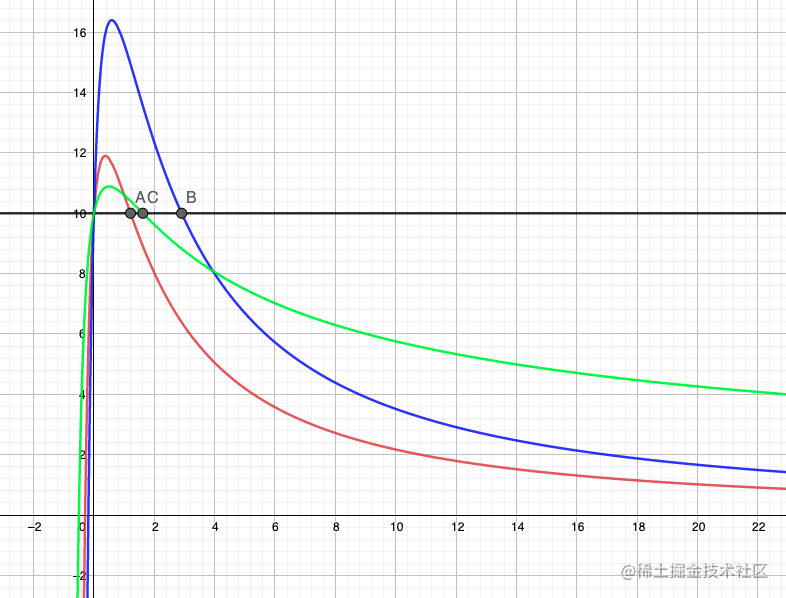• $A$$W=20\times{\log_{2}{(T+1)}}$$I=10$的交点
• $B$$W=20\times{\log_{1.5}{(T+1)}}$$I=10$的交点
• $C$$W=20\times{T}$$I=10$的交点

## 内容热度在掘金里的使用

$H = \frac{W + I}{(T+1)^{G}}$

• $H$：即一个内容的热度 rankIndexArticle
• $W$：即一个内容的 hotIndexArticle 是一个文章阅读数、评论数、点赞数加权求和的数值
• $I$：即一个内容初始的数值 rankIndexUser 是文章作者的影响因子
• 影响因子与作者本身的历史掘力值相关
• $G$：一个衰减的重力参数
• $T$：文章自发布以来的时长

• $c$：一篇文章本身的质量表达，多快获得点赞、获得阅读，即点击率、点赞率
• $d$：点赞率、点击率随着文章存在时间是否会衰减，衰减得多块

### 不同问题下的参数调整

• 新内容太多，近期热门的不足
1. 降低初始数值 $I$
2. 降低衰减重力 $G$
3. 提高内容质量的加权数值 $c$
• 内容都是热门的，新东西看不到
1. 提高初始数值 $I$
2. 提高衰减重力 $G$
3. 降低加权 $c$
• 一会儿是一个老的热门的，一会儿是一个特别新的
1. $G$ 较小的同时，$I$ 过大